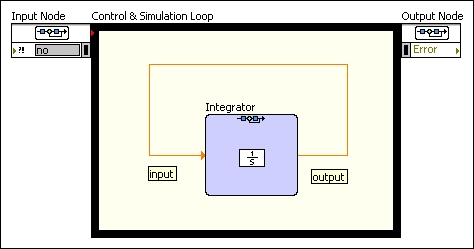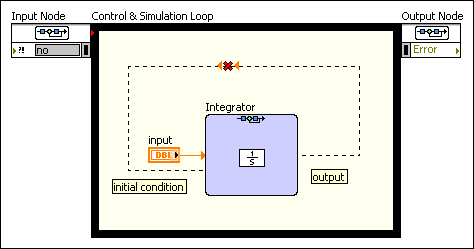# Determining Feedthrough Behavior and Defining Feedback Cycles (Control Design and Simulation Module)

LabVIEW 2018 Control Design and Simulation Module Help

Edition Date: March 2018

Part Number: 371894J-01

»View Product InfoDownload Help (Windows Only)

The relationship between a function input and output defines the feedthrough behavior of that I/O pair. An I/O pair can have indirect, direct, or parameter-dependent feedthrough behavior. If an I/O pair has indirect feedthrough behavior, you can create a feedback cycle between that input and output. An I/O pair with direct feedthrough behavior does not allow a feedback cycle. The following sections provide more information about these behaviors.

## Indirect Feedthrough and Feedback Cycles

Whereas LabVIEW VIs execute only after receiving the value of all inputs to that VI, many Simulation functions can execute without receiving the value of certain inputs. Consider a Simulation function with input u and output y. At any time step, if the function does not require the value of u to compute the value of y, u has indirect feedthrough to y.

When indirect feedthrough exists between u and y, you can create a feedback cycle between these parameters. In a feedback cycle, the value of y at time t relies on the value of u at time tdt, tdt2, and so on.

For example, the input parameter of the Integrator function has indirect feedthrough to the output parameter. You can create a feedback cycle between this input and output. The following simulation diagram shows this behavior:You can use one or more Simulation functions and other LabVIEW functions in a feedback cycle as long as at least one Simulation function in the feedback cycle has indirect feedthrough behavior. The indirect feedthrough function can start the data flow by executing the function output at the current step before receiving an input from the cycle at the current step. Therefore, the input at the current step and the output at the current step must not depend on each other directly in at least one function in the cycle.

The following Simulation functions have at least one I/O pair with indirect feedthrough.

## Direct Feedthrough

If a function output y requires an input u in order to execute, u has direct feedthrough to y. You cannot create a feedback cycle between inputs and outputs with direct feedthrough.

For example, the initial condition parameter of the Integrator function has direct feedthrough behavior to the output parameter. This function requires a value for the initial condition parameter in order to calculate the output parameter. Other functions, such as Friction, require the values of all inputs in order to execute.

If you attempt to create a feedback cycle between an input and output with direct feedthrough, the wire appears broken. The following simulation diagram shows this behavior:Notice the difference between the previous simulation diagram and the diagram showing the feedback cycle.

## Parameter-Dependent Feedthrough

Several functions have feedthrough behavior that depends on how you configure the parameters of that function. For example, consider the Transfer Function function. The feedthrough behavior of this function depends on the order of the numerator and denominator polynomial equations you specify. The following Simulation functions have at least one I/O pair with parameter-dependent feedthrough.

If you use the configuration dialog box to define the parameters of these functions, such as the numerator and denominator of a transfer function, LabVIEW automatically determines the appropriate feedthrough behavior and displays this choice in the Feedthrough pull-down menu. However, if you use block diagram terminals to define the parameters of these functions, you must set the feedthrough behavior manually.

All Simulation functions not mentioned in this section or in the Indirect Feedthrough and Feedback Cycles section have direct feedthrough.